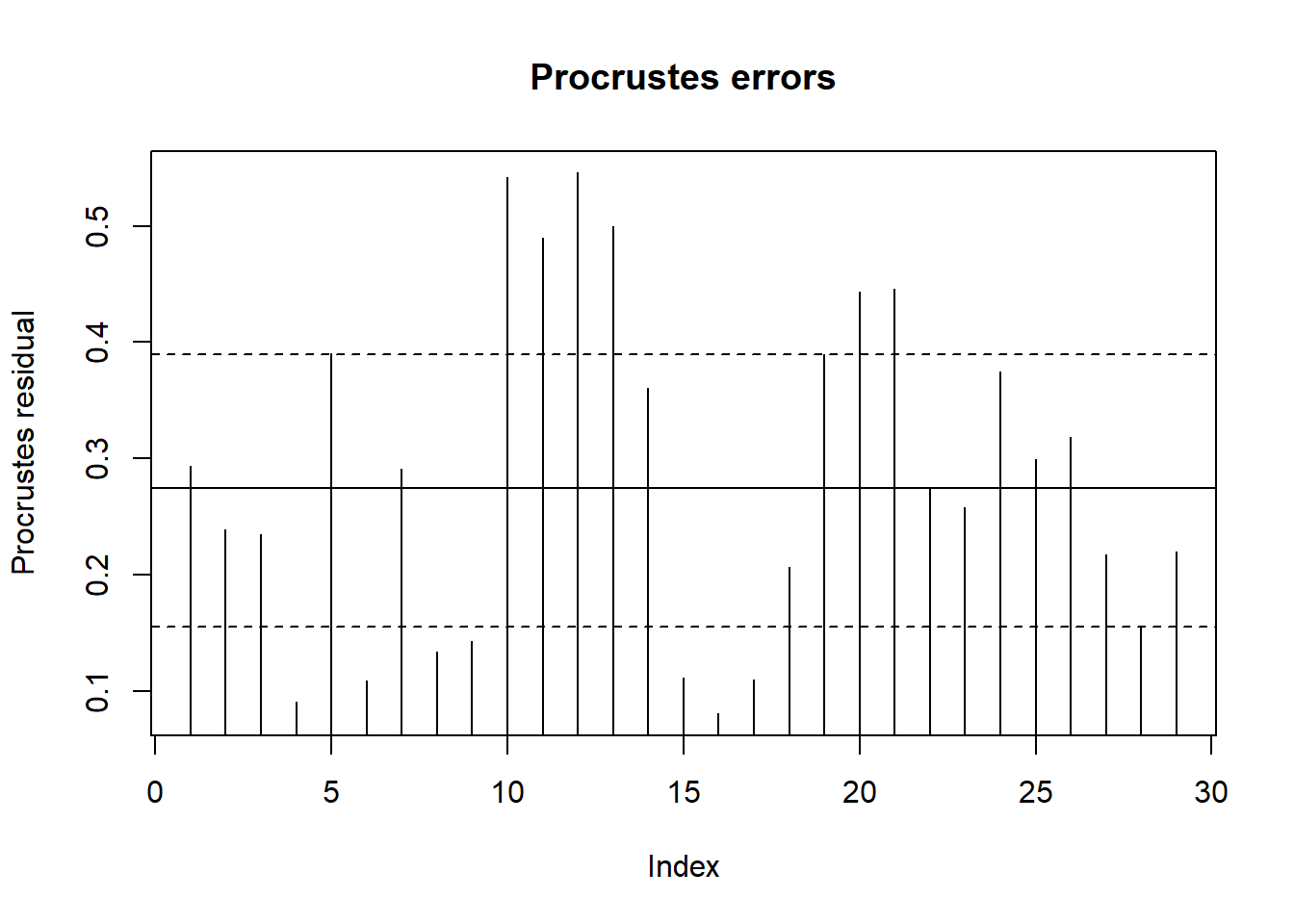# Procrustes Analysis

Procrustes analysis is the analysis of shapes. It takes its name from the bandit Procrustes (meaning “he who stretches”) in Greek mythology. Procrustes would offer travelers an evening meal and a night’s rest in his special bed – special because its length matched the height of anyone who lay on it. If the guest was too short, Procrustes would rack (stretch) him until he fit the bed. If the guest was too tall, Procrustes would cut off his legs to match the length of the bed. Needless to say, no one ever spent a second night in the bed. Procrustes met his end when the Greek hero Theseus adjusted him to fit the bed.

As microbial ecologists, and lacking such a bed and willing guests, our interest is generally in comparing different ordinations of our data. Think of ordinations as depicting the shape of our data in ordination space. We might compare ordinations based on environmental and species (OTU) data, for example, or between different methods of ordination. In “Multivariate Analysis of Ecological Communities in R: vegan tutorial,” Jari Oksanen compares ordinations by two NMDS methods.

Here I will compare ordinations of environmental and species data using the Doub’s River data from Numerical Ecology with R. The data are available for download from http://adn.biol.umontreal.ca/~numericalecology/numecolR/.

To follow along, first download the data and unzip it to your R working directory. Then load the `vegan` package and the data as in the following statements. You will have to edit the path to the data to correspond to your installation.

## Prepare Data and Ordinate

``````suppressPackageStartupMessages(library(vegan))
# Edit the path in the following line to match your installation.

There are no species present at site 8, so we need to remove that site from the data.

``````spe <- spe[-8, ]
env <- env[-8, ]``````

Next we need to standardize the environmental data to means of 0 and standard deviations of 1. Then we can calculate and plot a Principal Component Analysis (PCA) for the environmental data. PCA is based on Euclidean distances which the `rda` function calculates for us.

``````env.std = decostand(env, method = "standardize")
pca.env <- rda(env.std)
plot(pca.env, scaling = 1, display = "sites", type = "text",
main = "PCA for Environmental Data")``````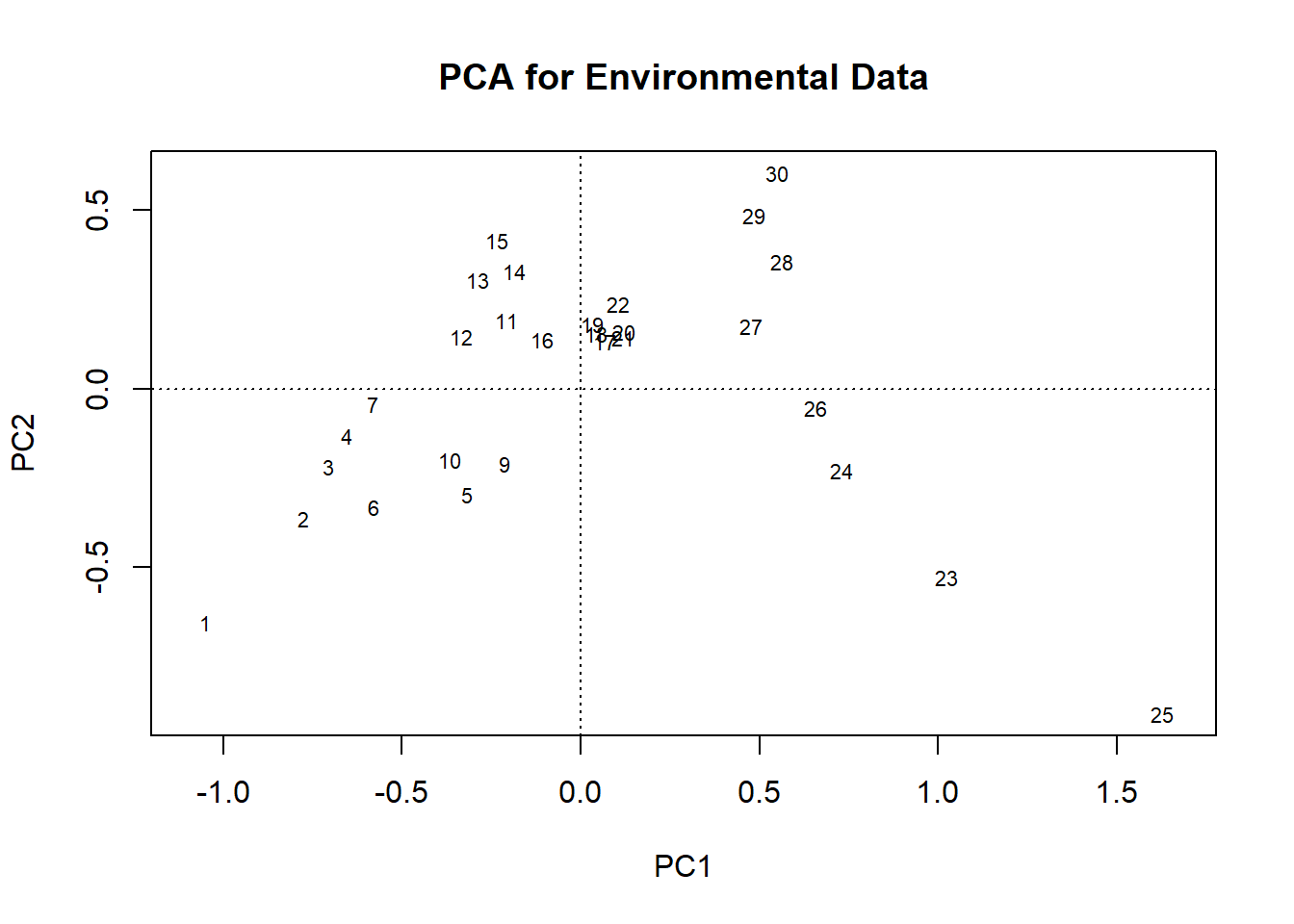PCA is not valid for species count data because it is based on Euclidean distances. But PCA can be applied to count data that has been Hellinger transformed (see Legendre and Gallagher, 2001). So next we transform the species data and calculate and plot the PCA for it.

``````spe.hel <- decostand(spe, method = "hellinger")
pca.spe <- rda(spe.hel)
plot(pca.spe, scaling = 1, display = "sites", type = "text",
main = "PCA for Species Data")``````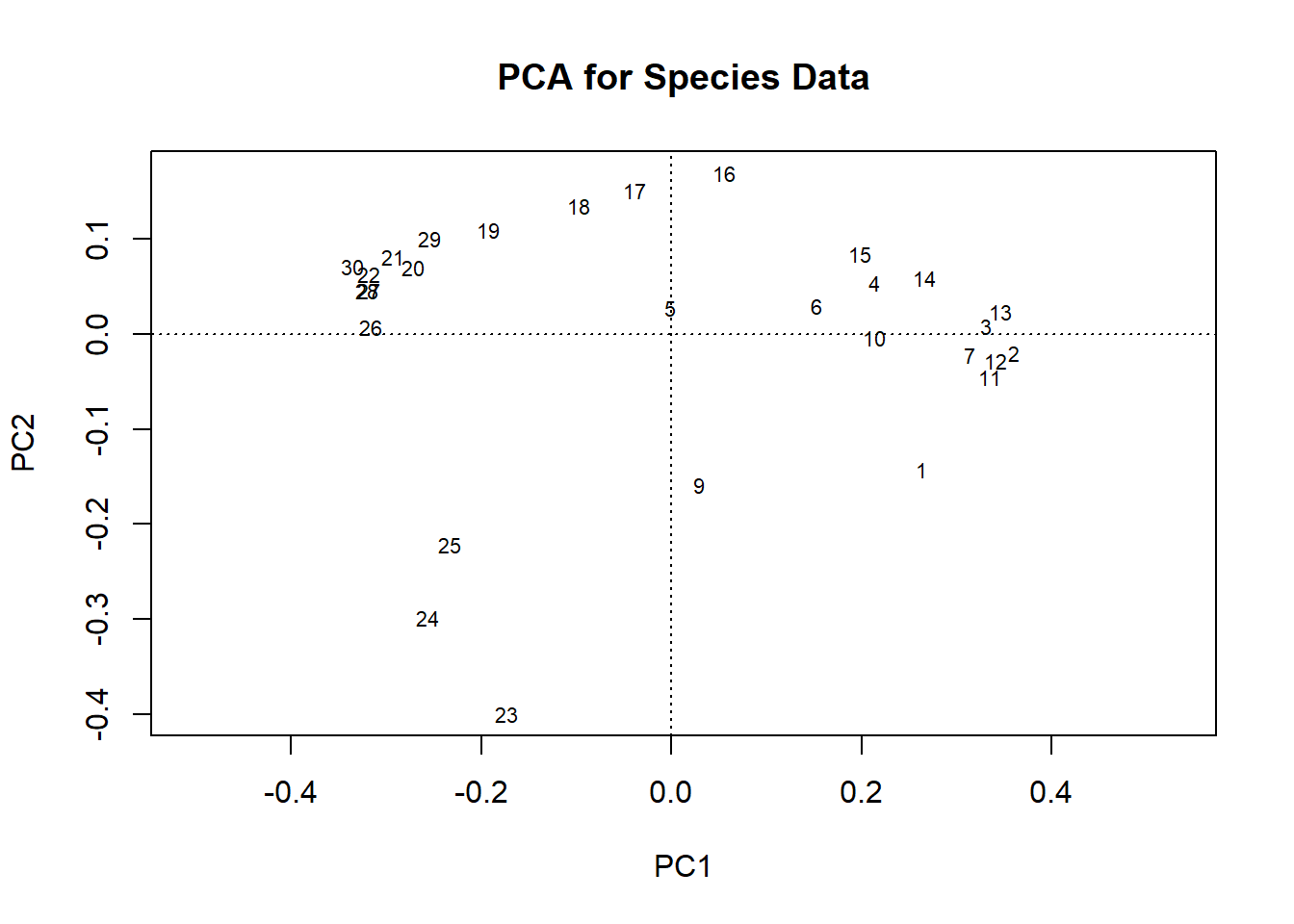## Procrustes Analysis

These ordinations do not look similar, but that could just be appearance. In performing a Procrustes analysis, the second ordination is rotated and usually re-scaled to minimize the sum of squared differences between the two ordinations. This makes the ordinations appear more similar. Think rotating and stretching, or shrinking, (but no chopping of legs!).

It makes more sense to put the PCA for the environmental data first because the species data is being fit to the environmental data, i.e. the target matrix. It is logical that species depend on the environment, but not the other way around. As we will see, however, the order makes no difference when making a test of significance.

``````pro <- procrustes(X = pca.env, Y = pca.spe, symmetric = FALSE)
pro``````
``````##
## Call:
## procrustes(X = pca.env, Y = pca.spe, symmetric = FALSE)
##
## Procrustes sum of squares:
## 14.18``````

By default, `symmetric` is FALSE, so I need not have included it the function call. But I did so to make a point. The analysis is not symmetric, but depends on the order of the ordinations. The print function for an object of class “procrustes” gives the function call and the sum of squares (i.e. sum of squared distances between paired points in the ordination space). Here it is 14.18. If we repeat `procrustes` with the order of the ordinations reversed, we get a different sum of squares.

``````pro <- procrustes(X = pca.spe, Y = pca.env, symmetric = FALSE)
pro``````
``````##
## Call:
## procrustes(X = pca.spe, Y = pca.env, symmetric = FALSE)
##
## Procrustes sum of squares:
## 3.031``````

If symmetric is set to TRUE, both solutions are first scaled to unit variance, giving a more scale-independent and symmetric statistic, often known as Procrustes m2. The order of the ordinations no longer matters.

``````pro <- procrustes(X = pca.spe, Y = pca.env, symmetric = TRUE)
pro``````
``````##
## Call:
## procrustes(X = pca.spe, Y = pca.env, symmetric = TRUE)
##
## Procrustes sum of squares:
## 0.4041``````
``````pro <- procrustes(X = pca.env, Y = pca.spe, symmetric = TRUE)
pro ``````
``````##
## Call:
## procrustes(X = pca.env, Y = pca.spe, symmetric = TRUE)
##
## Procrustes sum of squares:
## 0.4041``````

Note: The value of `symmetric` affects only the statistic calculated. It does not affect the rotation of Y.

## Plotting Results

There are two kinds of procrustes plots in `vegan`. Kind 1 gives a visual indication of the degree of match between the two ordinations. Symbols or labels show the position of the samples in the first ordination, and arrows point to their positions in the target ordination. The plot also shows the rotation between the two ordinations necessary to make them match as closely as possible.

``plot(pro, kind = 1, type = "text")``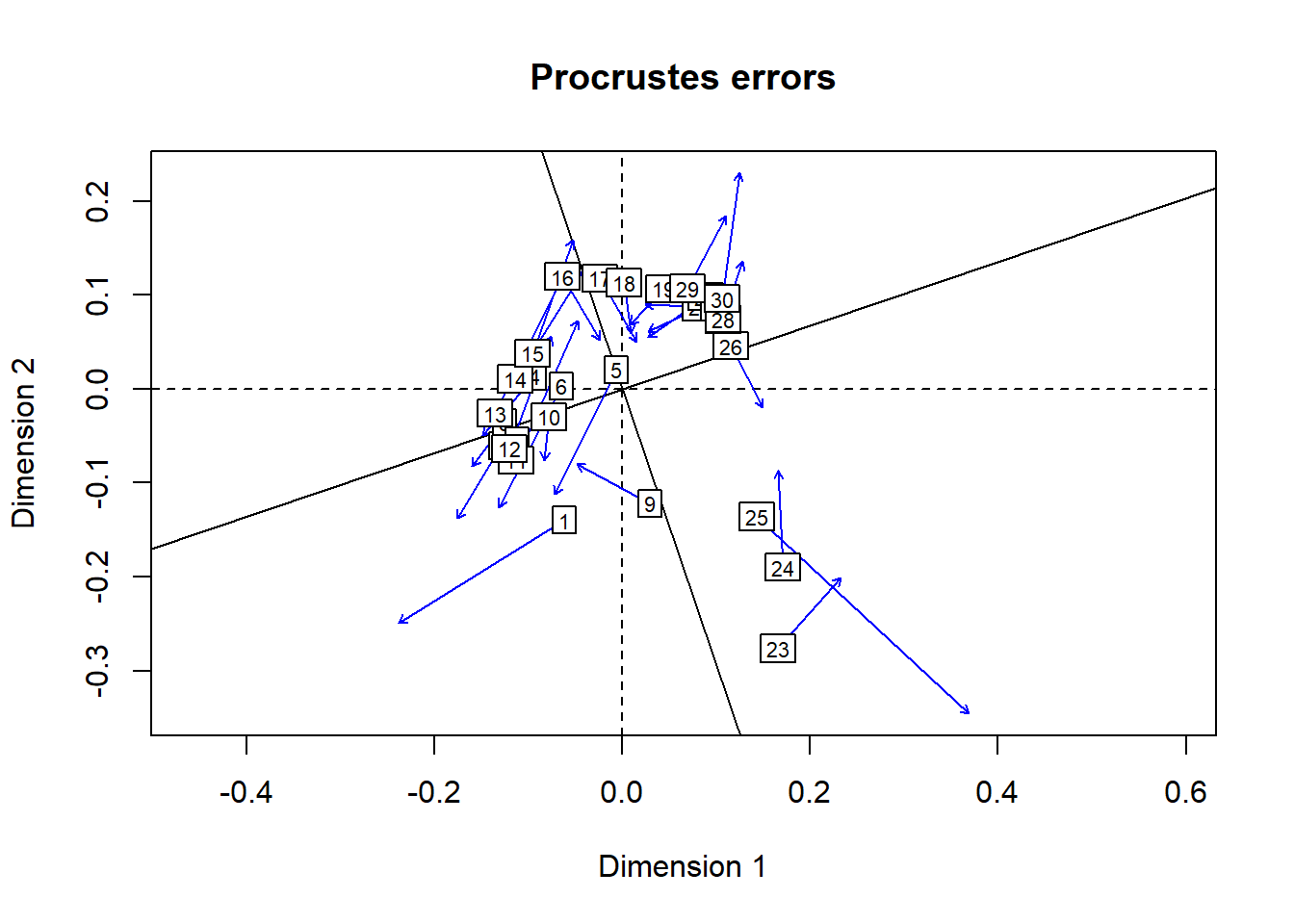Kind 2 plots show the residuals for each sample. This allows identification of samples with the worst fit. The horizontal lines, from bottom to top, are the 25% (dashed), 50% (solid), and 75% (dashed) quantiles of the residuals.

``plot(pro, kind = 2)``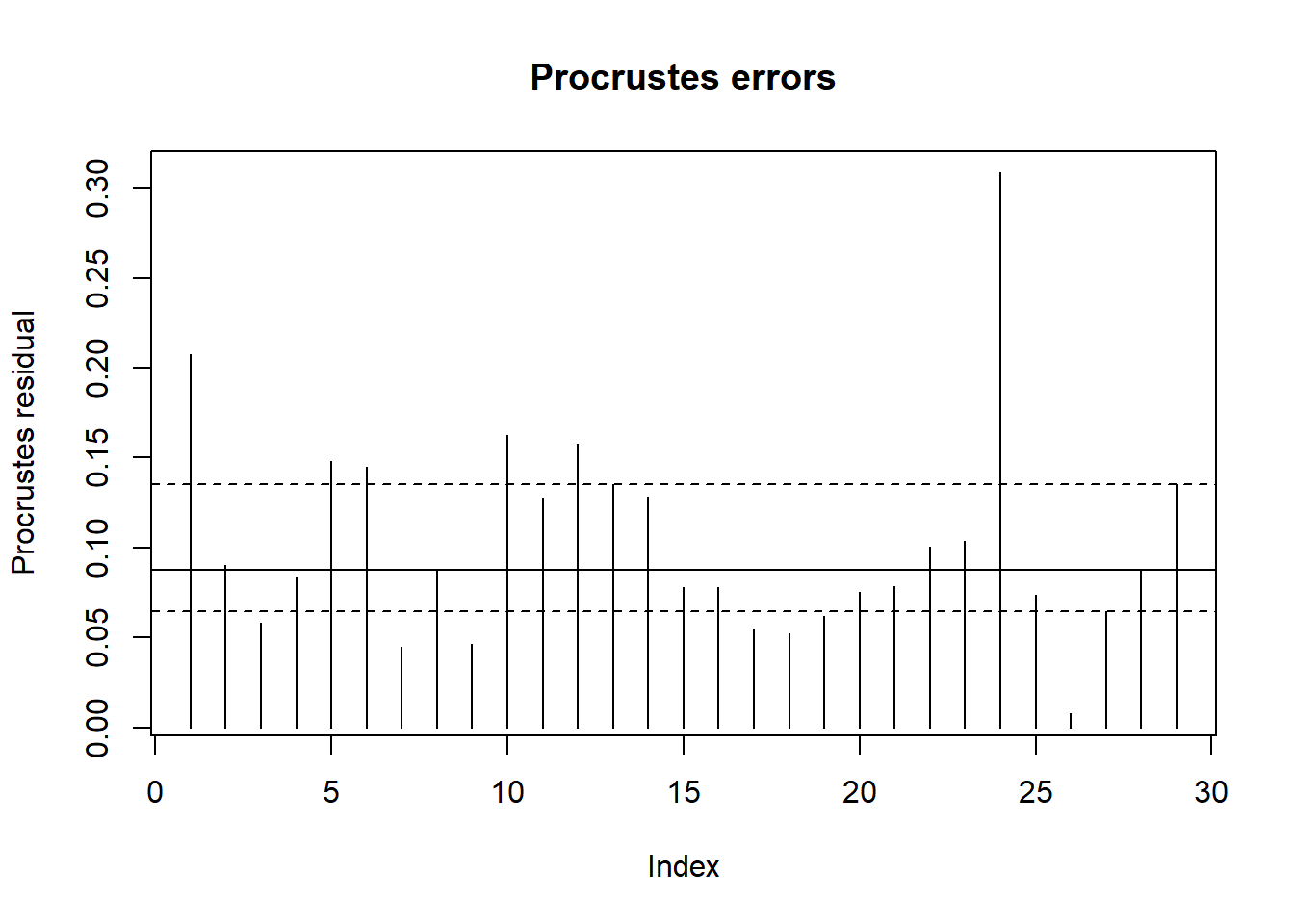## Test of Significance

Function `protest` is a permutational test of the significance of the procrustes result. It is based on the correlation from a symmetric Procrustes analysis. This is why the order of X and Y makes no difference to the test of significance.

``protest(X = pca.env, Y = pca.spe, scores = "sites", permutations = 999)``
``````##
## Call:
## protest(X = pca.env, Y = pca.spe, scores = "sites", permutations = 999)
##
## Procrustes Sum of Squares (m12 squared):        0.4041
## Correlation in a symmetric Procrustes rotation: 0.772
## Significance:  0.001
##
## Permutation: free
## Number of permutations: 999``````

In this case, the kind 1 plot is a bit messy, but the `protest` result indicates that there is a significant correlation between the two ordinations.

Note: This is a permutation test. The significance cannot be less than 1/(permutations + 1). I limited the number of permutations to 999 to save run time. If I had used a larger number, the reported significance would likely be much smaller. Try it yourself and see.

## Other Ordination Methods

Procrustes analysis can be applied to other ordination methods as long as `vegan` can extract scores from the results. If X has fewer axes than Y, however, we will get a warning that one of the ordinations has been adjusted. This adjustment involves adding extra columns of zeroes to the matrix of scores extracted from X so that it has the same number of columns as the matrix of scores extracted from Y. This is necessary to allow Y to be rotated to match X, but is not always desirable. To prevent using matrices of unequal dimensions, it may be necessary to specify the axes in the function call.

For example, we can perform a Principal Co-ordinates Analysis (PCoA) for the species data using Bray distances. PCAs are not calculated from Bray distances but only from Euclidean distances. PCoAs can be calculated from any distance matrix. In the code below I use the` is.euclid` function from the `ade4` package to determine if the distance matrix is euclidean in the sense of not generating negative eigenvalues. If it is not, then the `add = add` argument to the` cmdscale` function will add a small value to all distances to prevent negative eigenvalues. This is called the Cailliez correction (Cailliez, 1983).

``````spe.bray <- vegdist(spe, method = "bray")
pcoa.spe <- cmdscale(spe.bray, k = nrow(spe)-1, eig = TRUE, add = add)
ordiplot(pcoa.spe, type = "text", main = "PCoA for Species Data, Bray Distances")``````
``## species scores not available``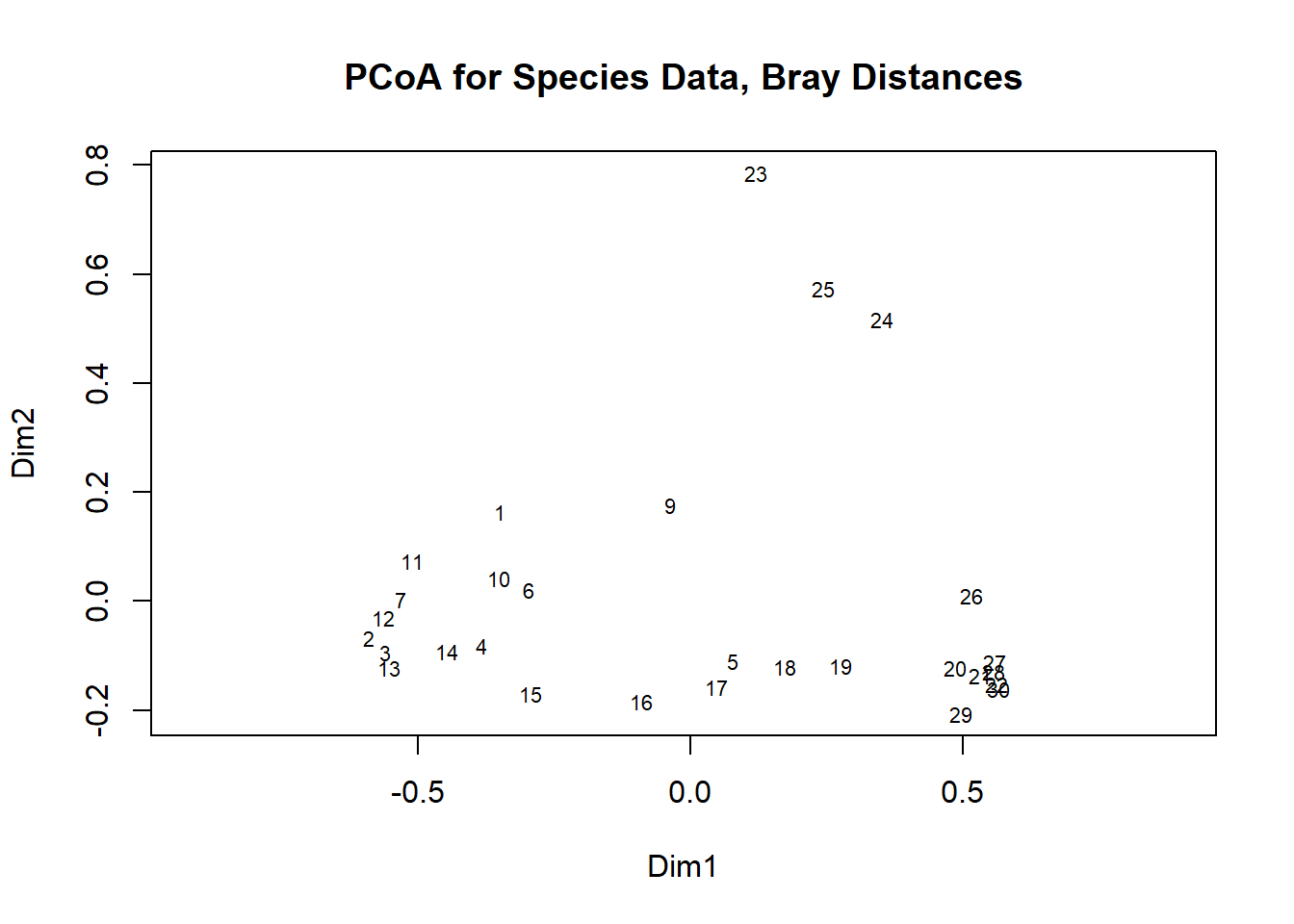``pro.1 <- procrustes(pca.env,pcoa.spe,  symmetric = TRUE, scores = "sites")``
``## Warning in procrustes(pca.env, pcoa.spe, symmetric = TRUE, scores = "sites"): X has fewer axes than Y: X adjusted to comform Y``
``pro.1``
``````##
## Call:
## procrustes(X = pca.env, Y = pcoa.spe, symmetric = TRUE, scores = "sites")
##
## Procrustes sum of squares:
## 0.6405``````

The warning is because of the way scores are extracted from the two ordinations. By default, `scores` extracts scores for only the first two axes from a PCA result, but for all available axes from a PCoA result. In this example, there are 28 axes for the PCoA result. You can prevent the warning by restricting the analyses to only the first two axes; just add `choices = c(1,2)` to the function call. For example:

``````pro.1 <- procrustes(pcoa.spe, pca.env, symmetric = FALSE, scores = "sites",
choices = c(1,2))
pro.1``````
``````##
## Call:
## procrustes(X = pcoa.spe, Y = pca.env, symmetric = FALSE, scores = "sites", choices = c(1, 2))
##
## Procrustes sum of squares:
## 2.921``````

Plots are made in the usual way.

``plot(pro.1, kind = 1)``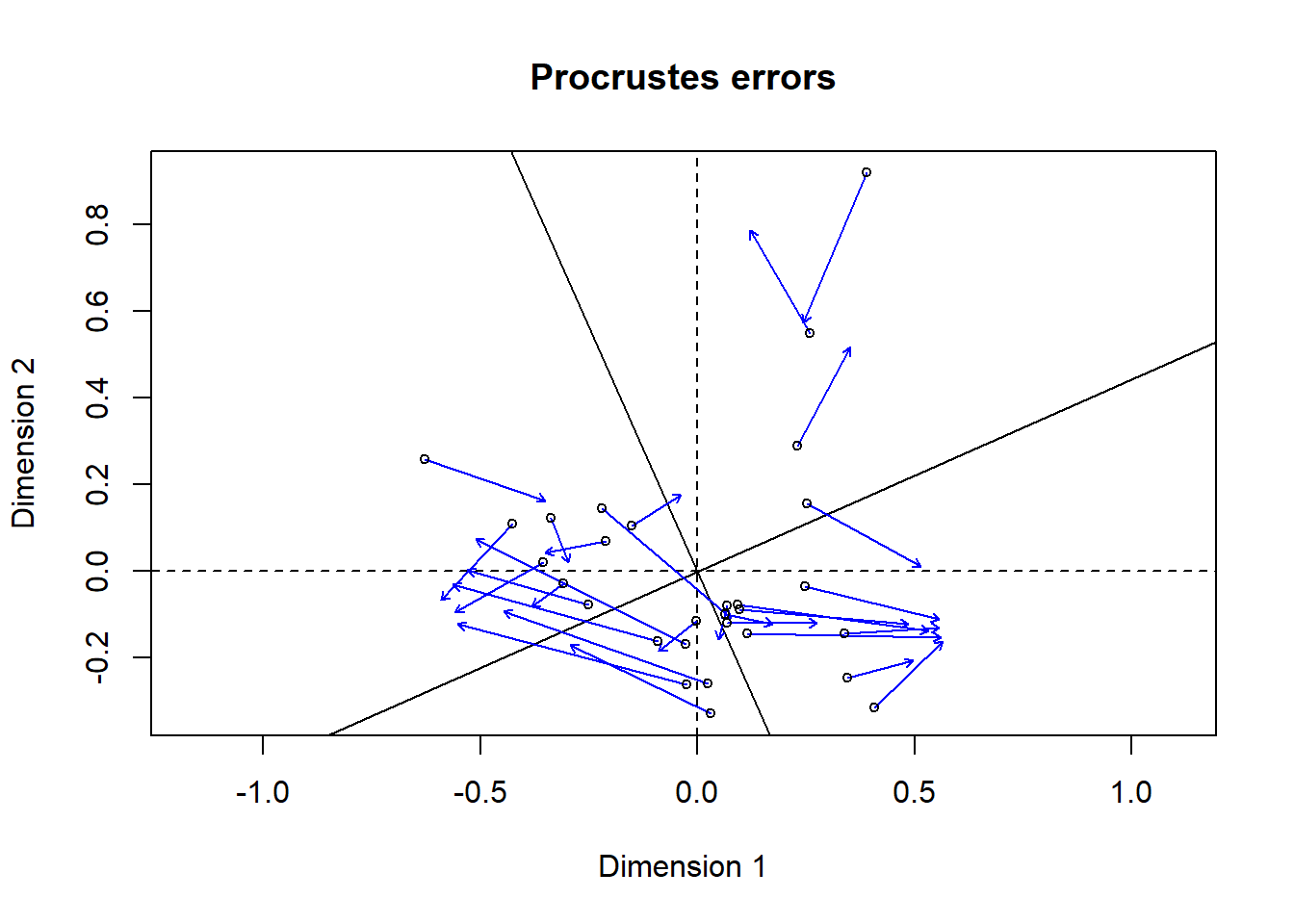``plot(pro.1, kind = 2)``